Transformations
Angle Pairs/ Triangle Congruence
Similarity
Right Triangles
100

A scale factor that would result in a similar figure with a smaller area.

-1<x<1

100

1. Angles 1 and 4 are ____________.

2. Angles 2 and 6 are ____________.1. vertical

2. corresponding

100

Select all that are true about the diagonals of rectangles:

A. perpendicular

B. congruent

C. bisect each other

D. bisect angles

B, C- B proves that a quadrilateral is a rectangle.

100

ABC ~ DEF. x = ____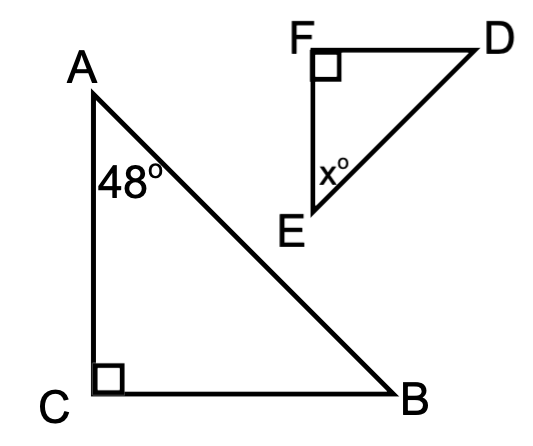42

100

sin(A) = ______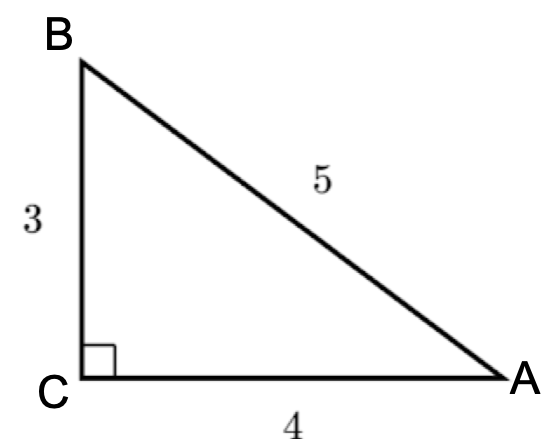3/5

200

The scale factor that takes A(-12, 24) to A'(-3, 6).

1/4 or .24

200

The triangles are congruent by the _____ Theorem.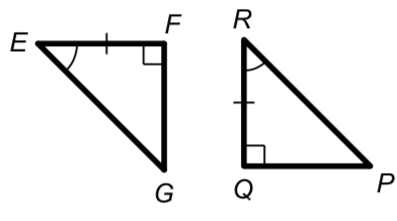ASA

200

c = ____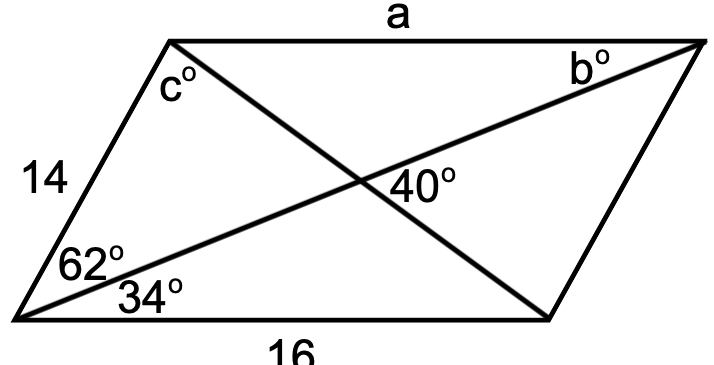78

200

EF = _____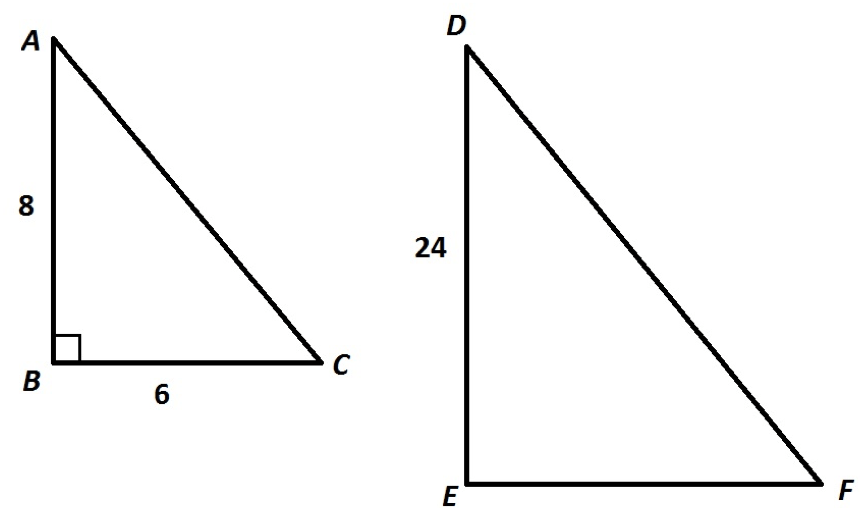18

200

In radical form, AT = ____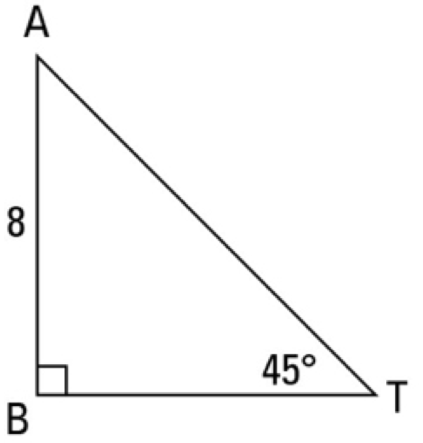8 root 2

300

1. The vector the translates (8, -2) to (4, 3).

2. The transformation that takes (6, -7) to (-6, 7). (not a translation)

1. <-4, 5>

2. 180 rotation

300

The triangles are congruent by the ____ TheoremAAS

300

a = ____, b = _____, and c = _____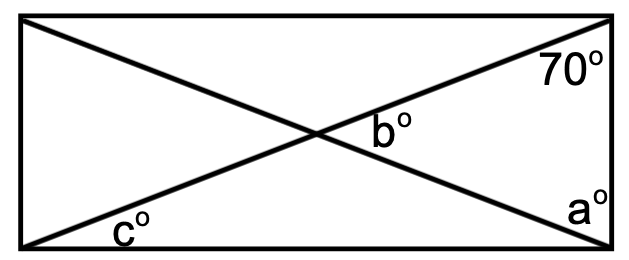a = 70 , b = 40, and c = 20

300

x = ____ Round to the tenths.3.4

300

The function used to find theta is ______.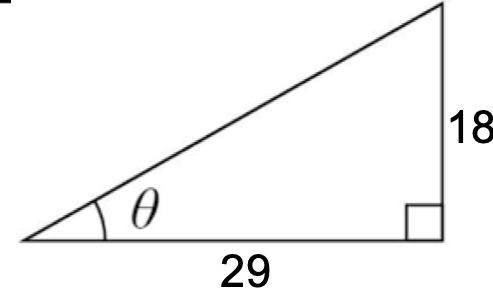tan-1

400

1. What is the image of (4, -5) after a reflection across the line y = x?

2. Which transformation takes (9, 3) to (3, -9)? (not a translation)

1. (-5, 4)

2. 270 degree ccw rotation

400

Fill in the blank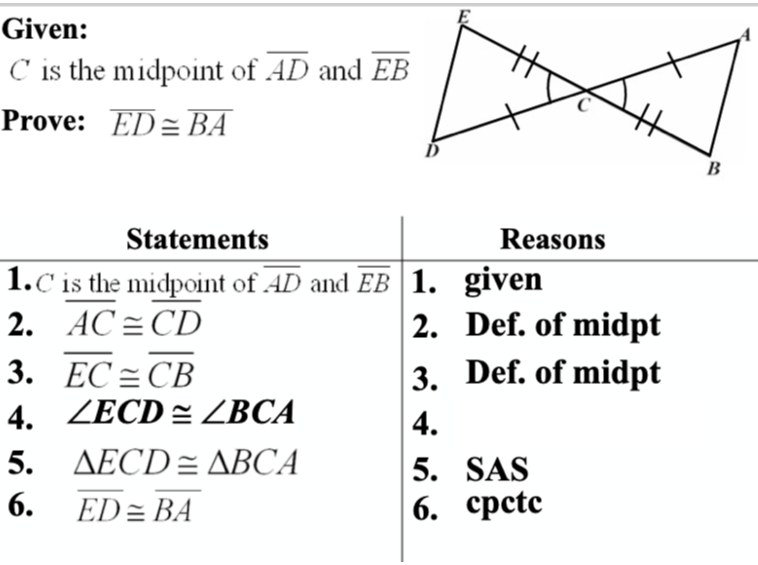Vertical Angle Theorem

400

Find x in the rectangle below.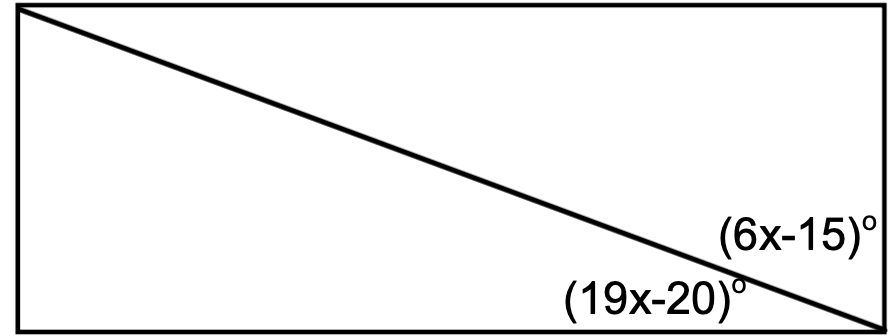5

400

A 400 foot building casts a 50 foot shadow. How tall is a nearby tree that casts a 5 foot shadow?

40 feet

400

a = _____12

500
What is the image of (7, -10) after a 270 degree ccw rotation and then a reflection across the y-axis?

(10, -7)

500

Fill in the blank.SAS

500

Solve for x in the parallelogram below.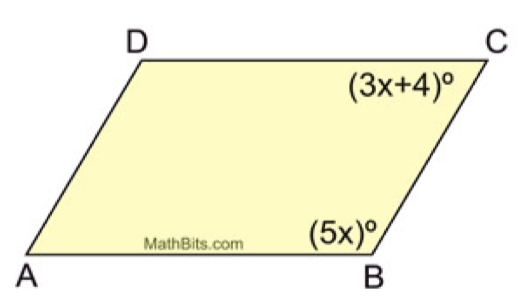22
500

x = ____ Round to the tenths place.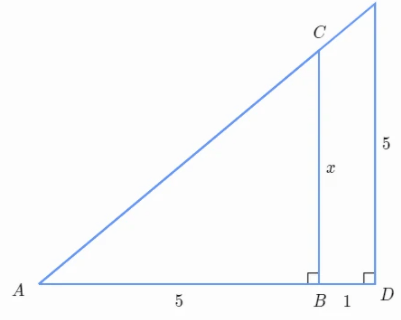4.2

500

x = ____ round to the tenths.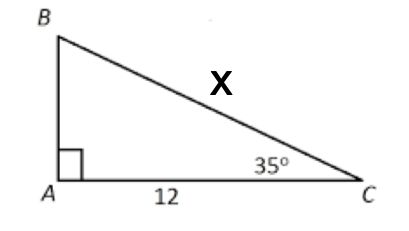14.6

Click to zoom